# ML Aggarwal Class 10 Solutions for ICSE Maths Chapter 17 Mensuration Ex 17.3

## ML Aggarwal Class 10 Solutions for ICSE Maths Chapter 17 Mensuration Ex 17.3

ML Aggarwal Class 10 Solutions for ICSE Maths Chapter 17 Mensuration Ex 17.3

Question 1.
Find the surface area of a sphere of radius:
(i) 14 cm
(ii) 10.5 cm
Solution: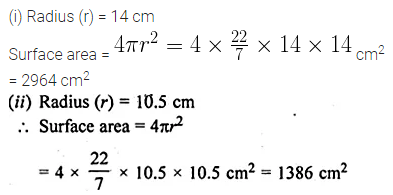Question 2.
Find the volume of a sphere of radius:
(i) 0.63 m
(ii) 11.2 cm
Solution:Question 3.
Find the surface area of a sphere of diameter:
(i) 21 cm
(ii) 3.5 cm
Solution:Question 4.
A shot-put is a metallic sphere of radius 4.9 cm. If the density of the metal is 7.8 g per cm3, find the mass of the shot-put.
Solution:Question 5.
Find the diameter of a sphere whose surface area is 154 cm2.
Solution:Question 6.
Find:
(i) the curved surface area.
(ii) the total surface area of a hemisphere of radius 21 cm.
Solution:Question 7.
A hemispherical brass bowl has inner- diameter 10.5 cm. Find the cost of tin-plating it on the inside at the rate of Rs 16 per 100 cm2.
Solution: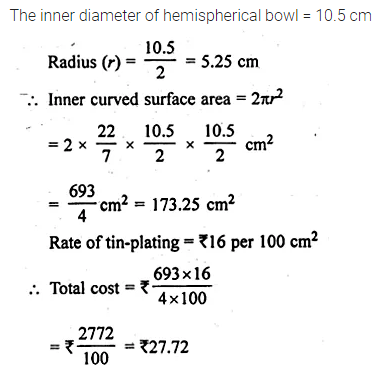Question 8.
The radius of a spherical balloon increases from 7 cm to 14 cm as air is jumped into it. Find the ratio of the surface areas of the balloon in two cases.
Solution: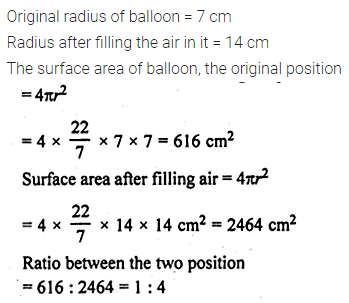Question 9.
A sphere and a cube have the same surface. Show that the ratio of the volume of the sphere to that of the cube is √6 : √π
Solution:Question 10.
(a) If the ratio of the radii of two sphere is 3 : 7, find:
(i) the ratio of their volumes.
(ii) the ratio of their surface areas.
(b) If the ratio of the volumes of the two-sphere is 125 : 64, find the ratio of their surface areas.
Solution: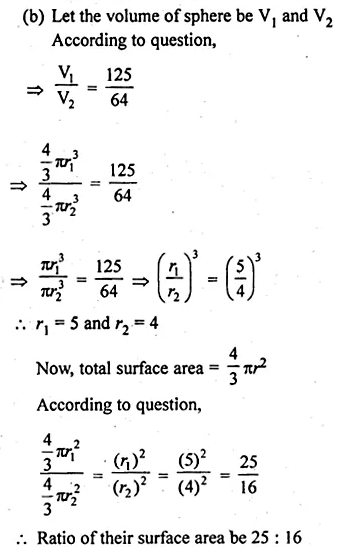Question 11.
A cube of side 4 cm contains a sphere touching its sides. Find the volume of the gap in between.
Solution: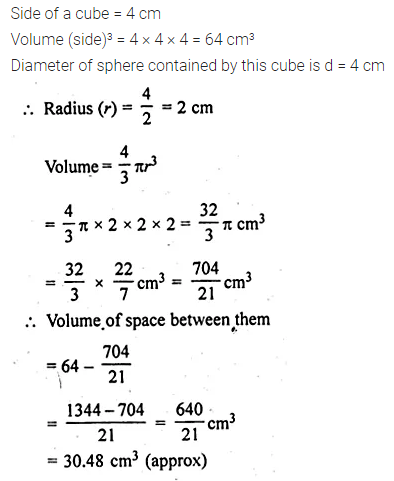Question 12.
Find the volume of a sphere whose surface area is 154 cm².
Solution:Question 13.
If the volume of a sphere is $$179 \frac { 2 }{ 3 }$$ cm³, find its radius and the surface area.
Solution:Question 14.
A hemispherical bowl has a radius of 3.5 cm. What would be the volume of water it would contain?
Solution:Question 15.
The water for a factory is stored in a hemispherical tank whose internal diameter is 14 m. The tank contains 50 kilolitres of water. Water is pumped into the tank to fill to its capacity. Find the volume of water pumped into the tank.
Solution: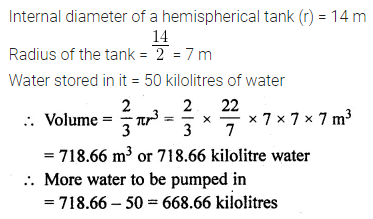Question 16.
The surface area of a solid sphere is 1256 cm². It is cut into two hemispheres. Find the total surface area and the volume of a hemisphere. Take π = 3.14.
Solution:Question 17.
Write whether the following statements are true or false. Justify your answer:
(i) The volume of a sphere is equal to two-third of the volume of a cylinder whose height and diameter are equal to the diameter of the sphere.
(ii) The volume of the largest right circular cone that can be fitted in a cube whose edge is 2r equals the volume of a hemisphere of radius r.
(iii) A cone, a hemisphere and a cylinder stand on equal bases and have the same height. The ratio of their volumes is 1 : 2 : 3.
Solution: